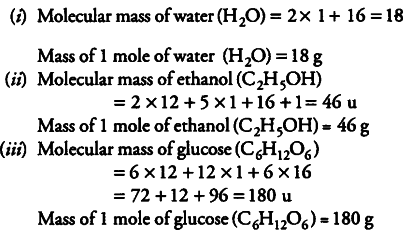# Find the mass of each one of the following

Find the mass of each one of the following:
(i) 1 mole of water
(ii) 1 mole of ethanol
(iii) 1 mole of glucose
[Atomic mass of H = 1 u, O = 16 u and C = 12 u]